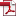• KSII Transactions on Internet and Information Systems
Monthly Online Journal (eISSN: 1976-7277)

# A Random Deflected Subgradient Algorithm for Energy-Efficient Real-time Multicast in Wireless Networks

Vol. 10, No. 10, October 30, 201610.3837/tiis.2016.10.013, Download Paper (Free):#### Abstract

In this work, we consider the optimization problem of minimizing energy consumption for real-time multicast over wireless multi-hop networks. Previously, a distributed primal-dual subgradient algorithm was used for finding a solution to the optimization problem. However, the traditional subgradient algorithms have drawbacks in terms of i) sensitivity to iteration parameters; ii) need for saving previous iteration results for computing the optimization results at the current iteration. To overcome these drawbacks, using a joint network coding and scheduling optimization framework, we propose a novel distributed primal-dual Random Deflected Subgradient (RDS) algorithm for solving the optimization problem. Furthermore, we derive the corresponding recursive formulas for the proposed RDS algorithm, which are useful for practical applications. In comparison with the traditional subgradient algorithms, the illustrated performance results show that the proposed RDS algorithm can achieve an improved optimal solution. Moreover, the proposed algorithm is stable and robust against the choice of parameter values used in the algorithm.